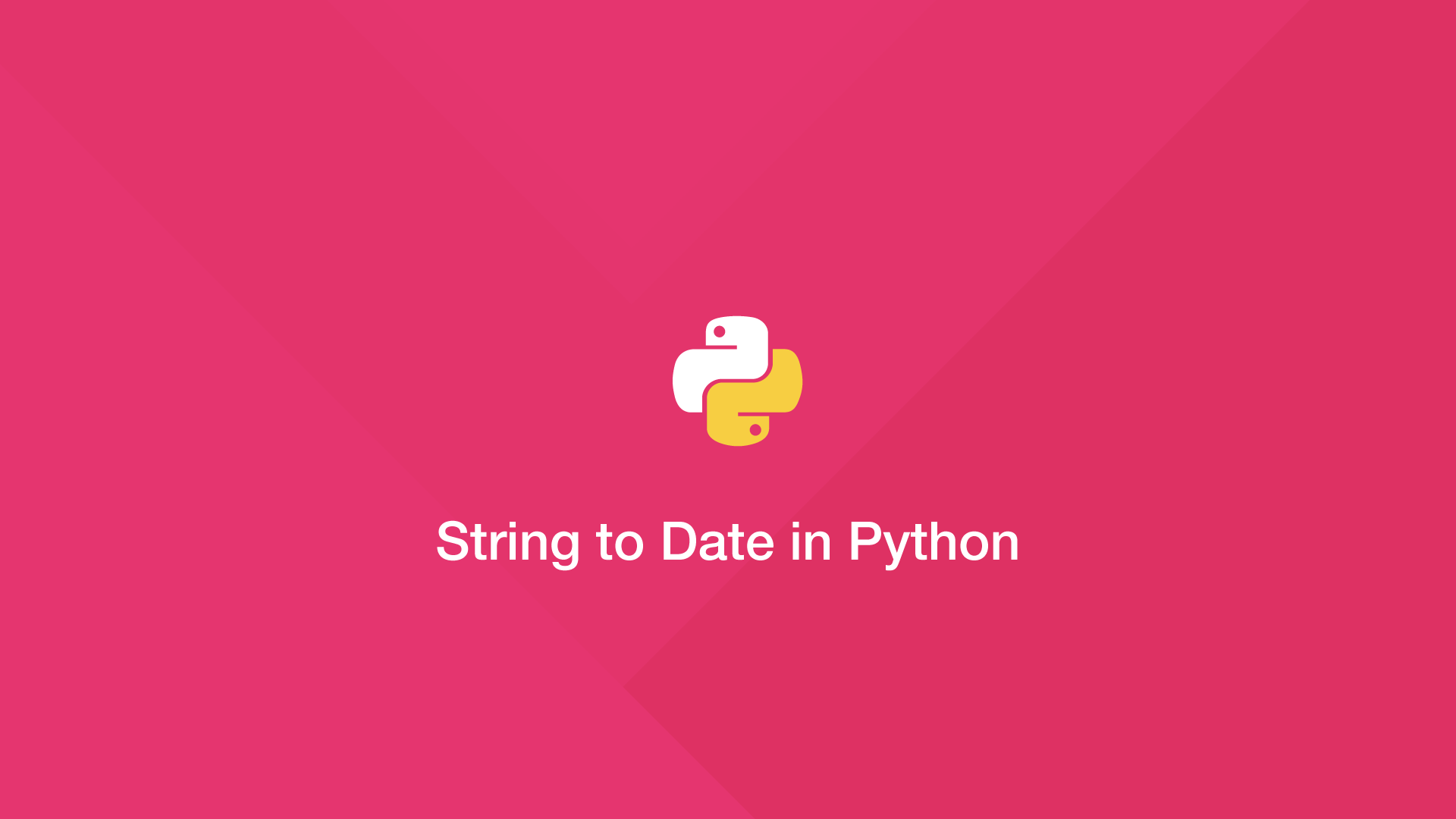# How to Convert a String to a Date in PythonTo convert a string to a date object in Python, import the `datetime` package and use the `striptime()` function. Pass the date to convert as the first argument and the format as the second.

Let's look at an example of changing a date string in a typical format to a Python date object.

``````from datetime import datetime

date_string = '28/07/2021 16:25:39'

date_object = datetime.strptime(date_string, '%d/%m/%Y %H:%M:%S')

print(date_object)
print(type(date_object))
``````
``````2021-07-28 16:25:39
<class 'datetime.datetime'>
``````

By wrapping the output in the Python `type()` function we can see the type class is now `datetime.datetime`.

The important thing to observe is that the input date string is formatted according to the format described in the second argument of `striptime()`. If these two are mismatched you will get a `ValueError` exception. Here an example to show this mistake:

``````date_string = '2021'

date_object = datetime.strptime(date_string, '%y')

print(date_object)
``````
``````ValueError: unconverted data remains: 21
``````

This happens because `%y` does not represent a four-digit year; `%Y` (uppercase) does, however.

``````from datetime import datetime

date_string = '2021'

date_object = datetime.strptime(date_string, '%Y')

print(date_object)
``````
``````2021-01-01 00:00:00
``````

#### Related Tutorials### How to use Python datetime to Create and use Dates and Times

September 24, 2020### Convert Unix Epoch/Timestamp to Datetime in Python

July 09, 2021### How to Format a Date in JavaScript

August 27, 2020### How to Convert DateTime to String in PHP

June 04, 2021### PHP DateTime - Create, Format and Compare Dates with PHP

September 04, 2020October 01, 2020